# How to Multiply in Google Sheets

Google Sheets provides its users with many ways to simplify any mathematical computation. People use them either to create a database or make simple calculations.

As multiplying can be one of the most essential functions in creating a balanced spreadsheet, in this article, we’ll tell you everything you need to know how to use it in Google Sheets. Furthermore, you’ll see how to create formulas to make your documents more efficient.

## How to Multiply in Google Sheets

When it comes to multiplying in Google Sheets, it’s a very straightforward process. Both approaches have the same principles, and you can use whichever one you find more suitable. The first way involves using a formula, and the second uses a multiply operand.

If you want to use a multiply formula, here’s what you need to do:2. Click on any place in the sheet and type “=multiply (<NUMBER1>, <NUMBER2>)” into the formula entry field using numerical values.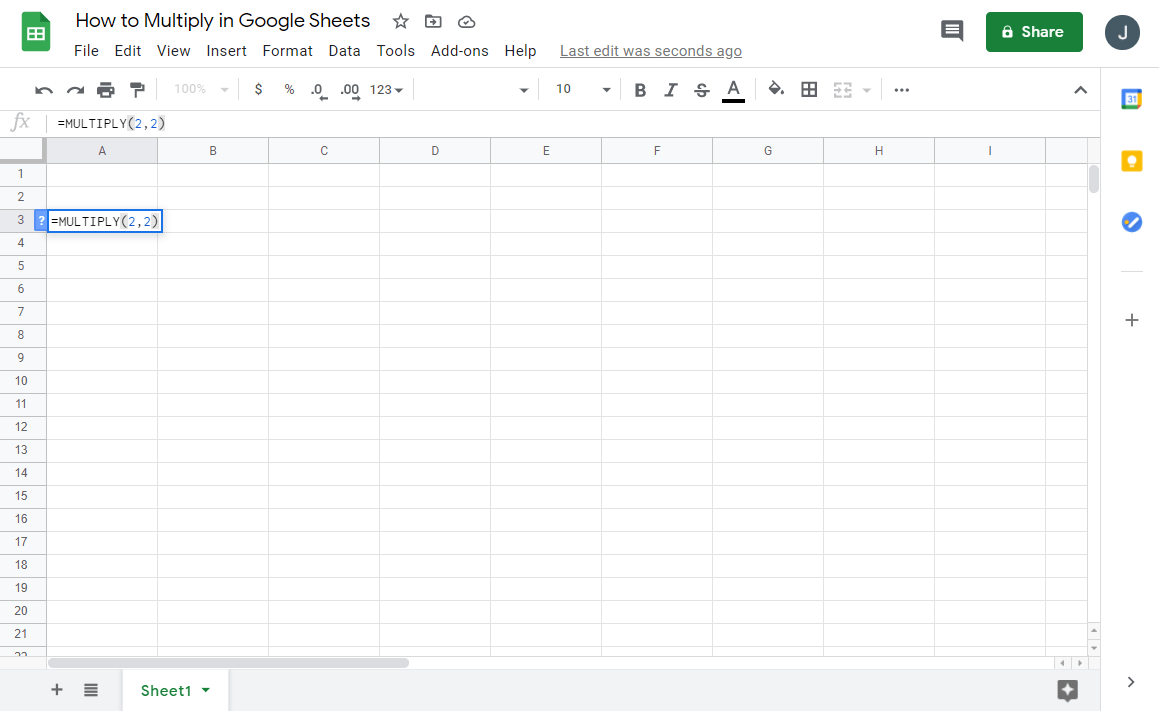3. Instead of numbers, you can use the cell code, and the spreadsheet will use its values.4. Once you press “Enter,” you’ll see the final value in the sheets.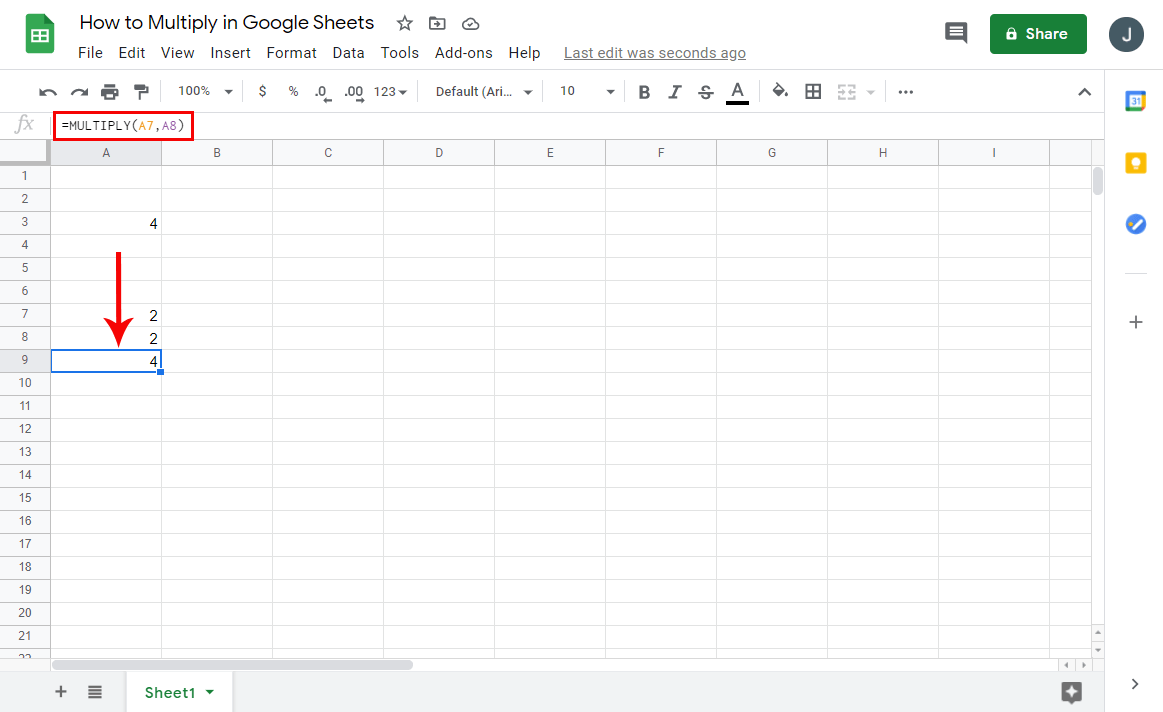Should you decide to use the multiply operand, its most significant upside is that you can use multiple numbers. Here’s how you can use the “*” symbol in Google Sheets:2. Click on any cell.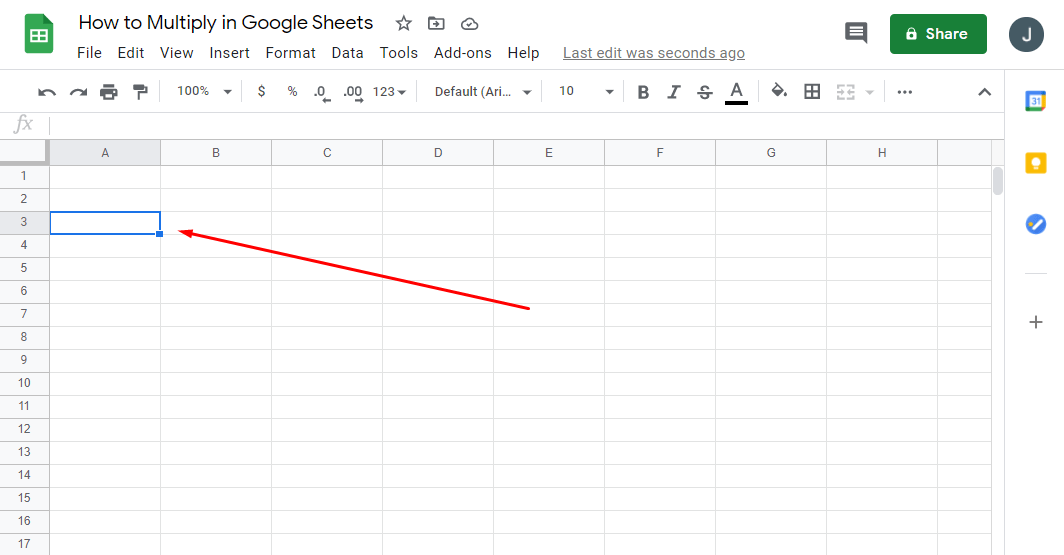3. Write “=<NUMBER1> * <NUMBER2>” in the formula field.4. Instead of numbers, you can write the cell code, and the spreadsheet will use its values.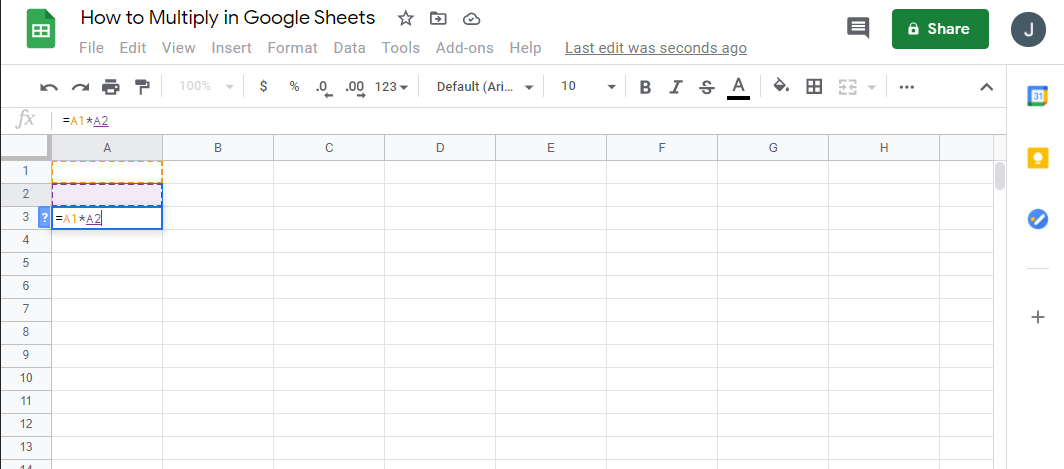5. Once you replace the numbers and press “Enter,” you’ll get the result.## Multiply Two Columns In Google Sheets

There are several formulas you can use for any mathematical expression. For multiplying two columns in Google Sheets, here’s what you need to do:2. If you have all your values in column A and column B, you’ll need to write the formula “=ARRAYFROMULA (A1:A12*B1:B12)”.3. Write the formula in a column other than A or B, and that column will display your results.When you’re using array formulas, it’s impossible to delete or edit a part of the result. However, the only thing you can delete is the whole array and set up a new one to give you a different result.

## Multiply an Entire Column in Google Sheets

In case you need to multiply an entire column in Google Sheets, and you’re not sure how to do it, simply follow these steps:3. Once you press “Enter,” you’ll see the final value in the column where you wrote the formula.## How to Multiply a Column by a Number in Google Sheets

If you have a column you wish to multiply by a number in Google Sheets, you can do it in a few straightforward steps. Here’s how:2. Write the number to multiply the whole column within the C1.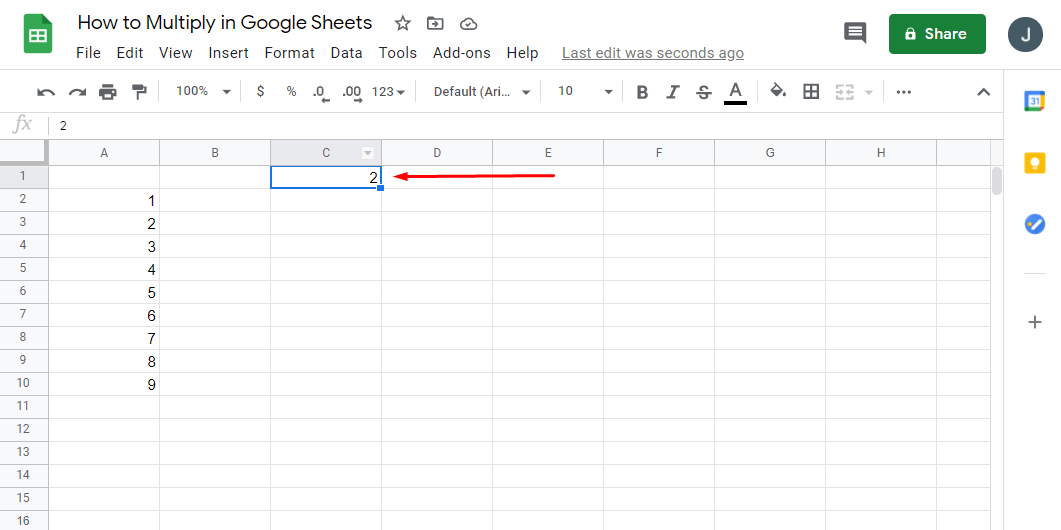3. Now, write this formula: “=A2*\$C\$1.”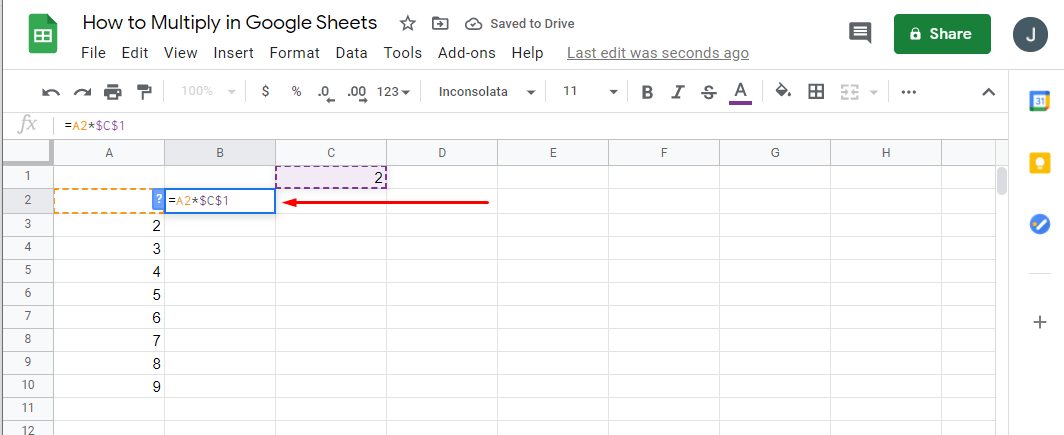4. To copy the formula through the column, press on the small square in the right cell corner and drag it to the column’s end.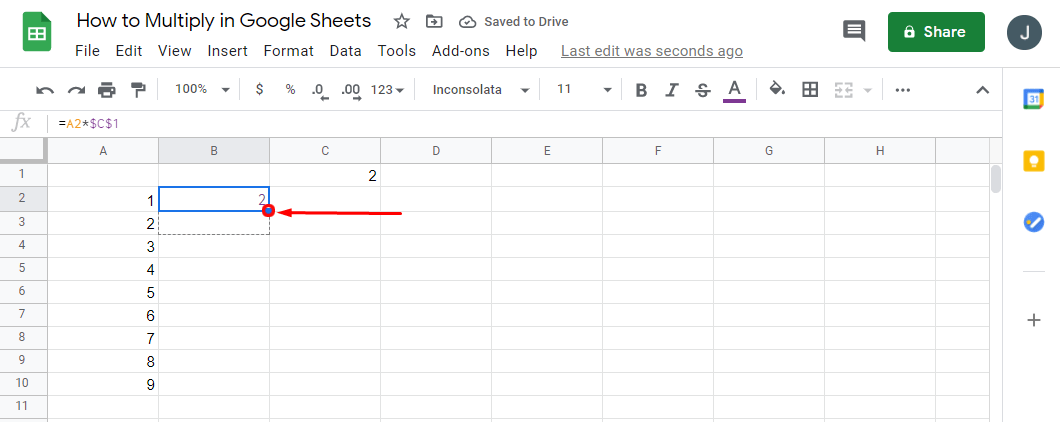5. Now the formula has been copied to all the fields, and you’ll see the results in column B.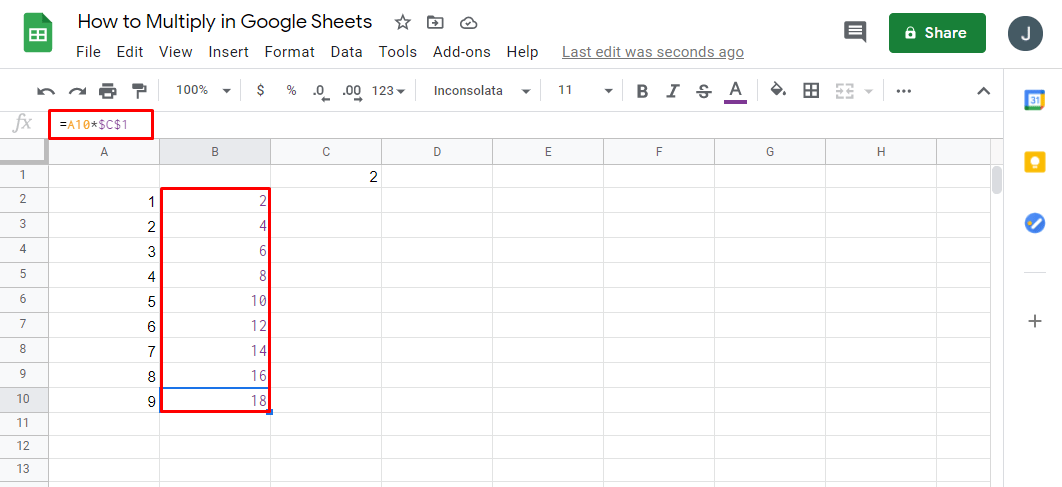## How to Multiply in Google Sheets Using Numbers, Cells, or Columns

There’s more than one way to multiply numbers in Google Sheets. As long as your formulas are correct, it’s of lesser importance to use cell names or numbers in the brackets. However, once your procedures start growing and spreadsheets get more data, it’s more efficient to use cell names.

There are several ways to get to the same solution:

1. Using a formula with numbers: “=MULTIPLY (1,2)”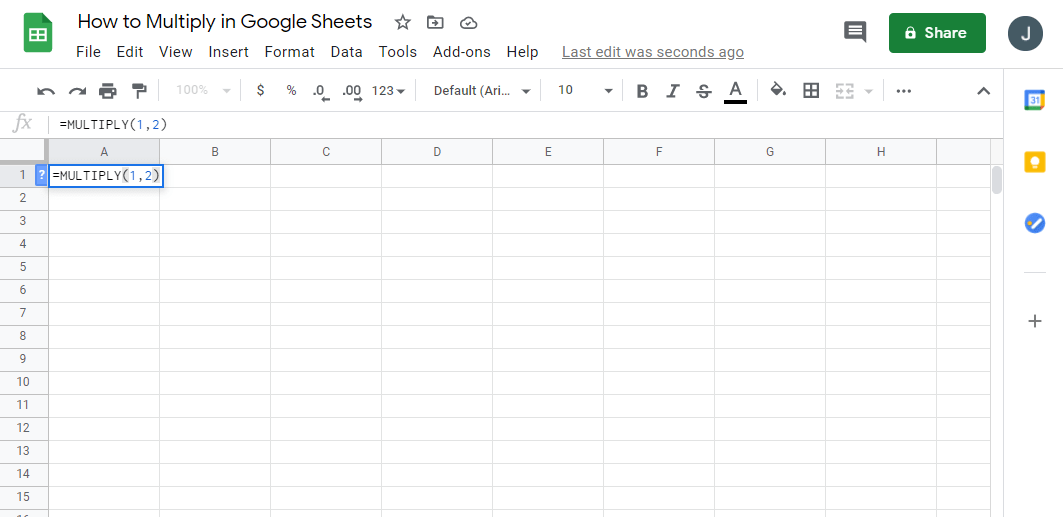2. Using a formula with cell names: “=MULTIPLY (A1, B2)”3. Using a multiply operator with numbers: “=2*3”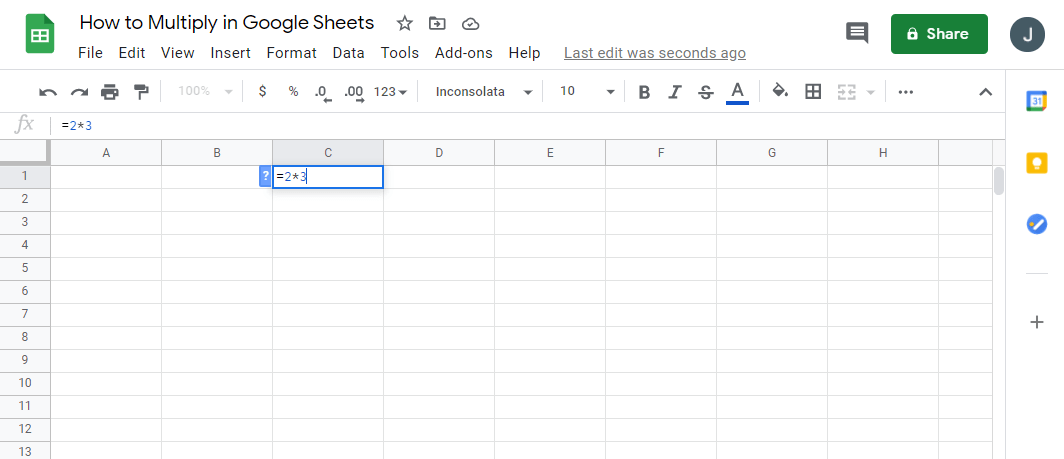4. Using a multiply operation with cell names: “=B1*B2”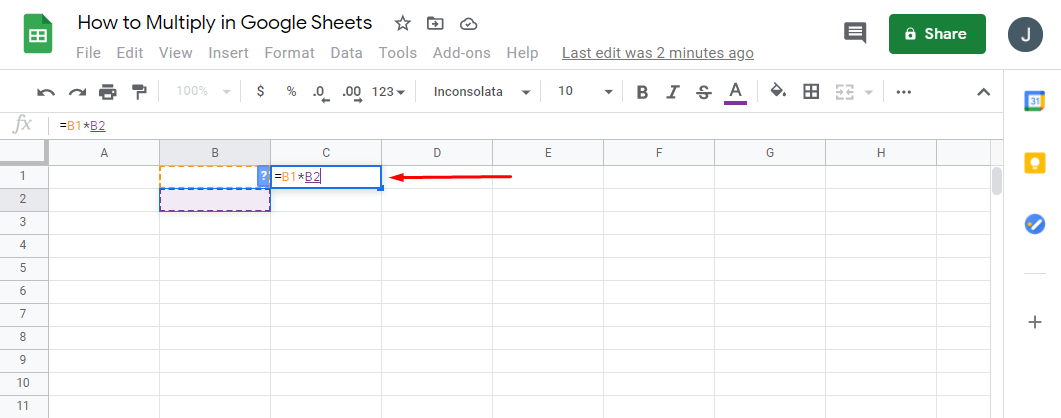### How Do You Multiply a Column by a Number in Sheets?

If you have a column that you need to multiply by a number in Google Sheets, you can do it in a few simple steps. Here’s how:• Write the number to multiply the whole column within the C1.• Now, write this formula: “=A2*\$C\$1.”• To copy the formula through the column, press on the small square in the right cell corner and drag it to the column’s end.• Now your formula has been copied to all the fields, and you’ll be able to see the results in column B.### How Do I Multiply Two Columns In Google Sheets?

Should you want two columns to multiply in Google Sheets, here’s what you need to do:• If you have numbers in column A and column B, you’ll need to write a formula: “=ARRAYFROMULA (A1:A12*B1:B12).”• It’s best to write this formula in cell C1 to fill the rest of the column’s cells with values.Using array formulas, you can’t delete or edit a part of the result, only the whole array.

### How Do I Multiply Multiple Cells in Google Sheets?

The best way to multiply multiple cells in your spreadsheets is to use the formula -“=A1*A2” – and add as many cells as you need. Using the array formula, you can multiply a large amount of data and create a new column with values. Here’s how to do it:• If you have columns A and B filled with information, you can choose column C to write the formula.• In C1, you can write “=ARRAYFORMULA ( * ).”• If your columns have a header, you should use “=ARRAYFORMULA (A2: AB2:B).”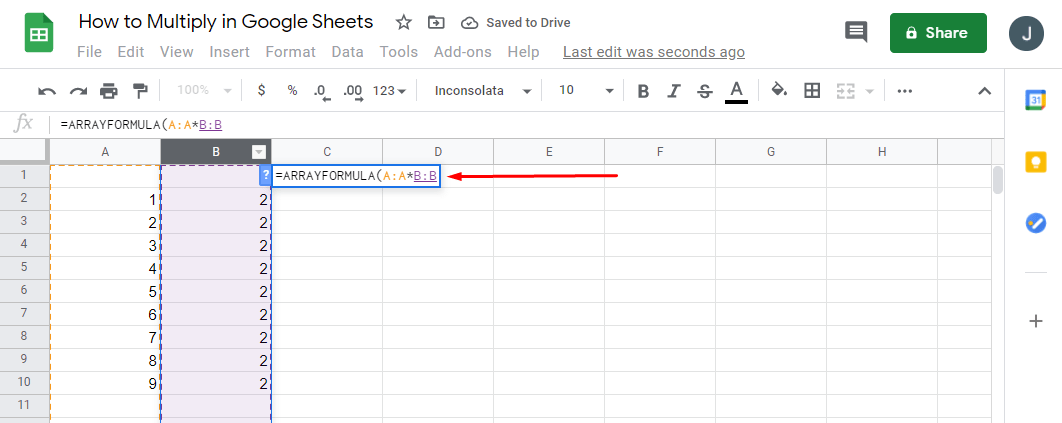If you want to select the range of fields to display the result, your formula should look like this: “=ARRAYFORMULA (A2:A20B2:B20).”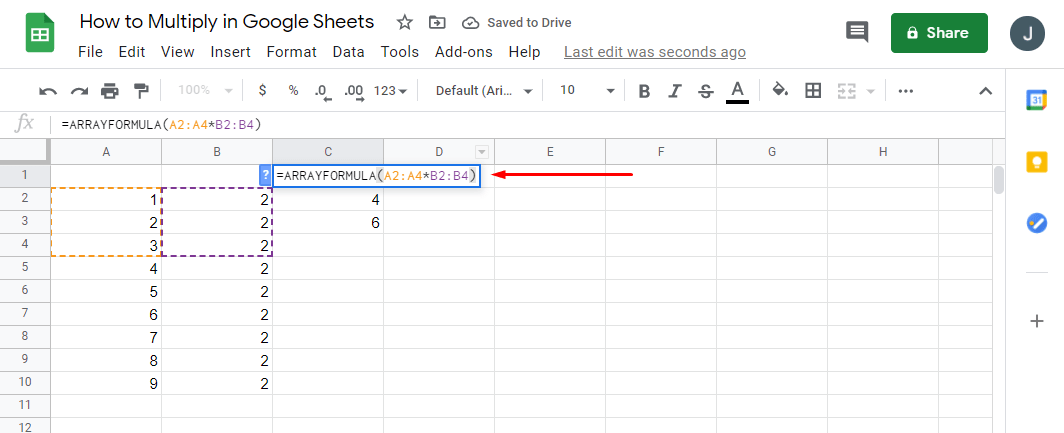### How to SUM Column in Google Sheets

SUM is one of the most fundamental processes in Google Sheets. Here’s how you can use the SUM column option in Google Sheets:• Highlight all the cells or a column you wish to calculate.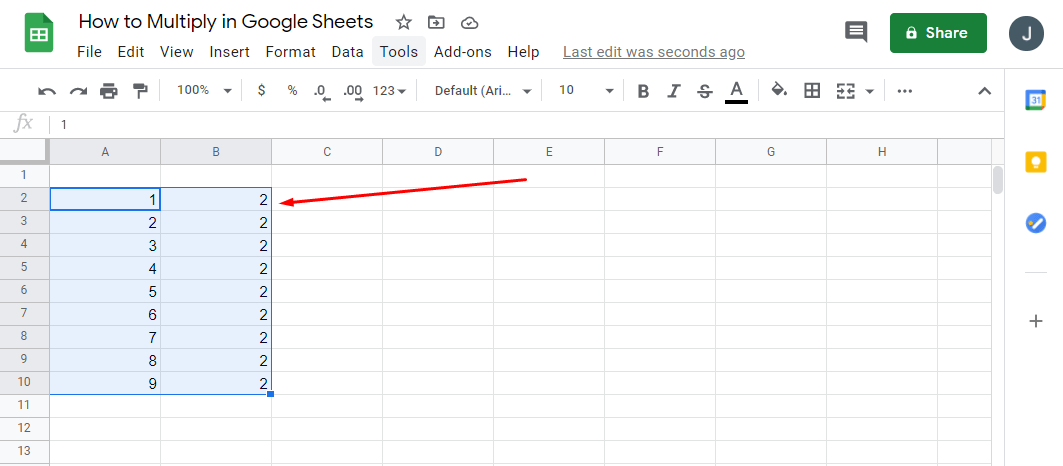• In the bottom right part of the screen, tap on “Explore” and “SUM: Total.”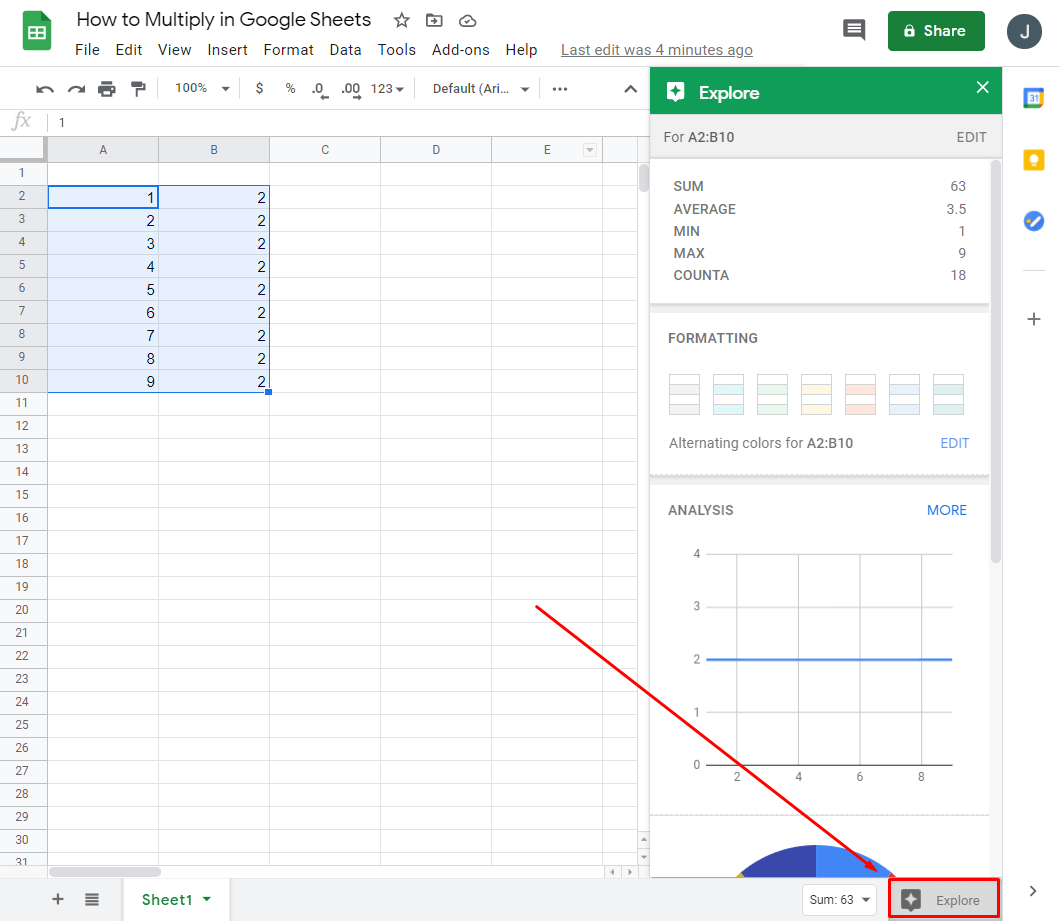• Should you tap on “SUM,” you’ll see more additional options.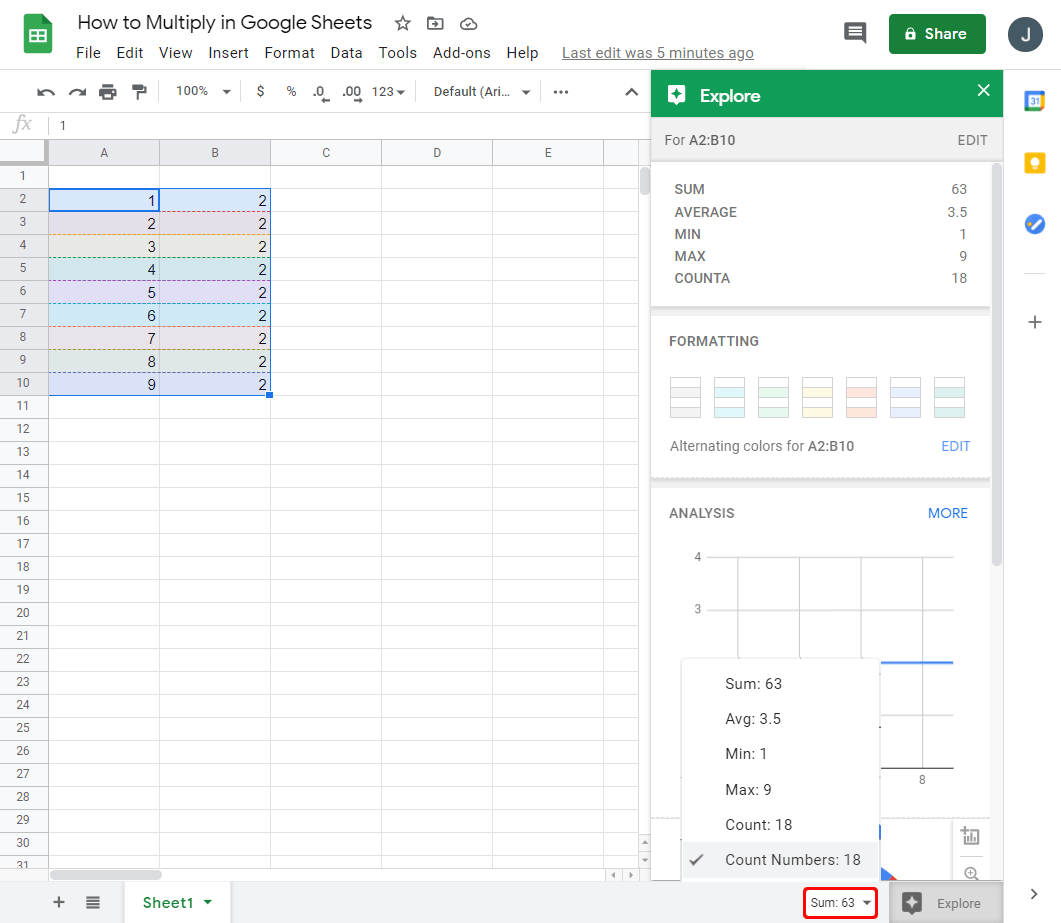Additional options that might be helpful are “average value,” “minimum,” and “maximum,” “count numbers,” or “count.” Keep in mind that unless you mark all the fields you want to be summed up, you won’t be able to see this option.

### How Do I Create a Formula in Google Sheets?

Using various formulas is one of the most important parts of Google Sheets. You can create them by tapping on any cell in the spreadsheet and then save them for other documents.

Every time you type “=” in any cell, you’re creating a function you want to use. Besides, you’ll often get suggestions depending on the formulas used to make sure you select the best one. Plus, there’s a function help box that’s always visible to provide you with useful definitions or formula syntax to help program the spreadsheet faster.

Once you start referencing other cells in the formula, they’ll automatically get highlighted, and they’ll appear in contrasting colors to differentiate them. This feature is especially handy when writing long expressions, and you need a clear perspective on how many different columns or cells that have been mentioned.

### How to Make Cell Bigger in Google Sheets

For specific projects, you might require cells to be larger than usual to create bigger Google Sheets for more data or comments. Here’s how to do it:• Select a row or a column you wish to change by holding a “Ctrl” and clicking on the cells or rows.• Right-click a column letter or a row number and choose between “Fit to Data” or “Enter a Custom Height or Width.”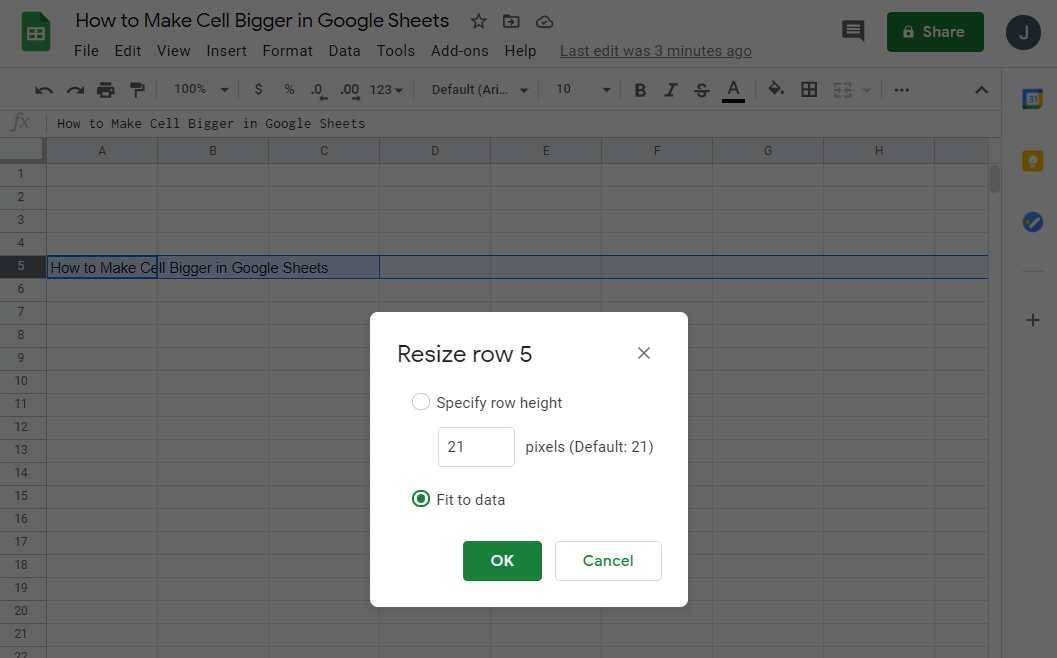• In the end, tap on “OK.”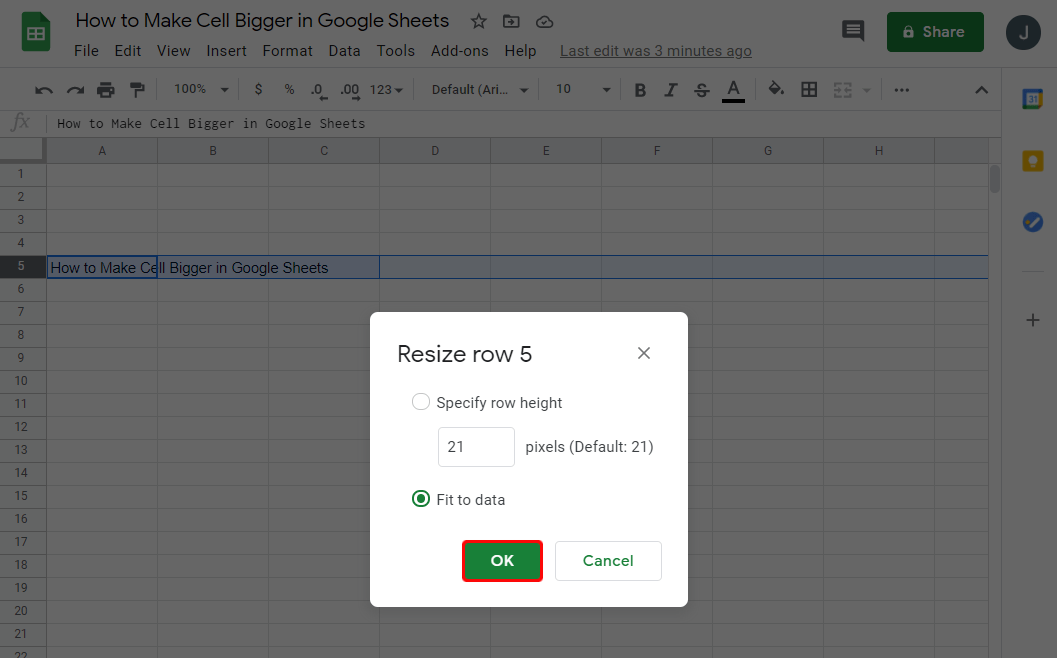### How Do You Multiply on a Spreadsheet?

There’s more than one way to multiply numbers in Google Sheets. You can use several ways to get to the same solution:

• Using a formula with numbers: “=MULTIPLY (1,2)”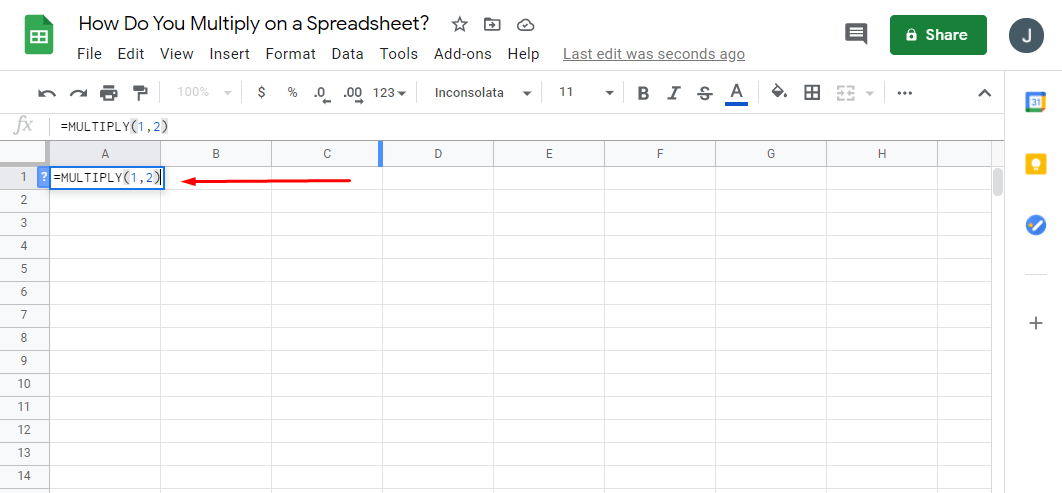• Using a formula with cell names: “=MULTIPLY (A1, B2)”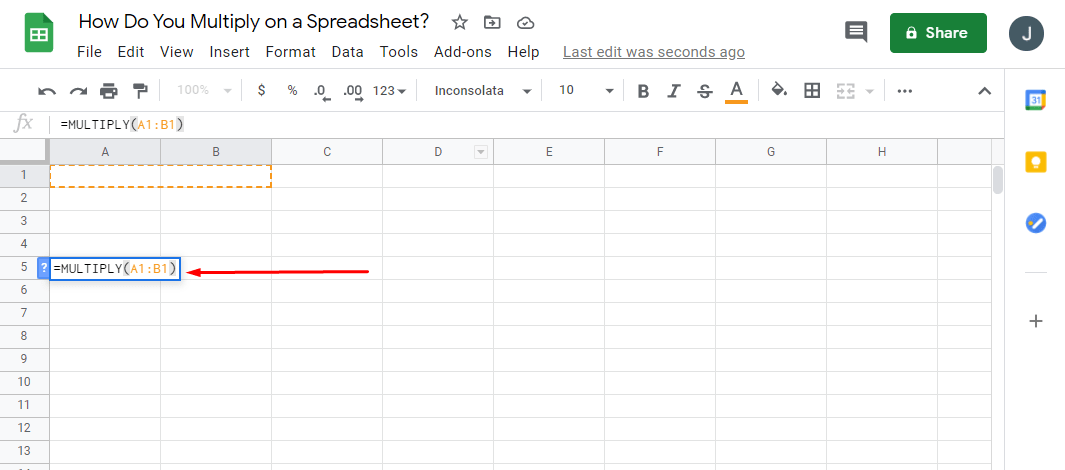• Using a multiply operator with numbers: “=23″Using a multiply operation with cell names: “=B1B2”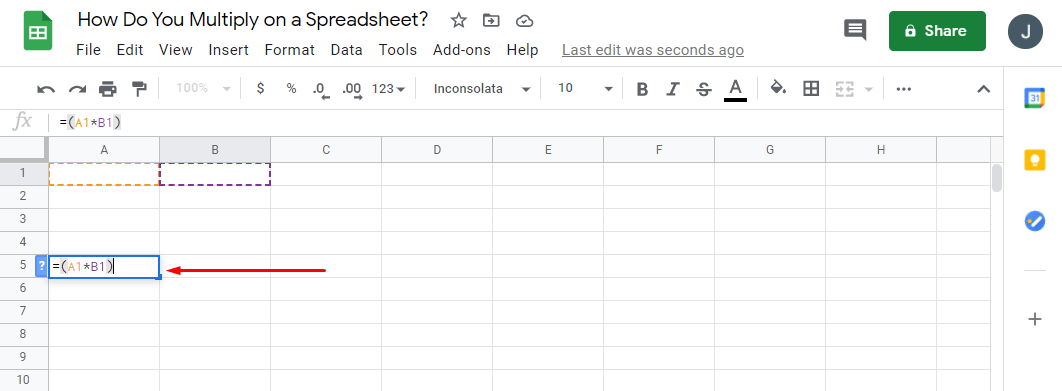### How to Divide Numbers in Google Sheets

As simple as it is to multiply, you can also divide numbers easily in Google Sheets. The only difference is the formula used and the operator. Here’s how to divide in Google Sheets:

• Using a formula with numbers: “=DIVIDE (1,2)”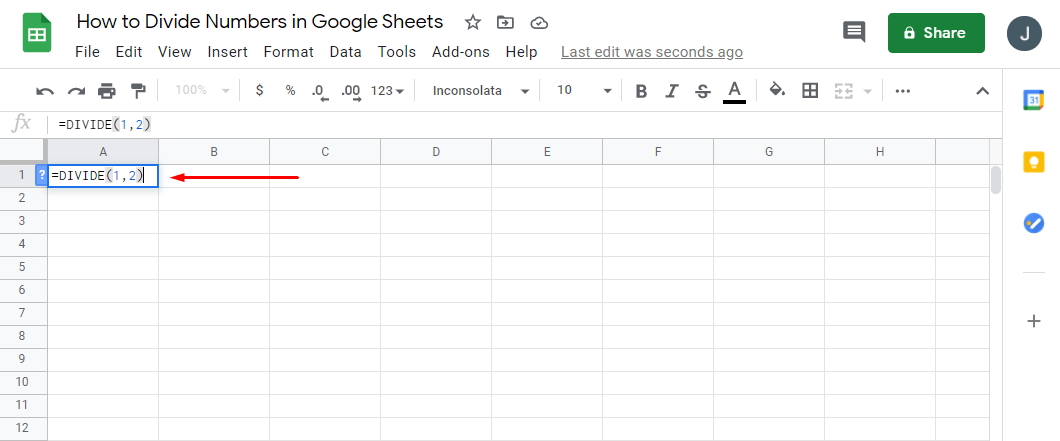• Using a formula with cell names: “=DIVIDE (A1, B2)”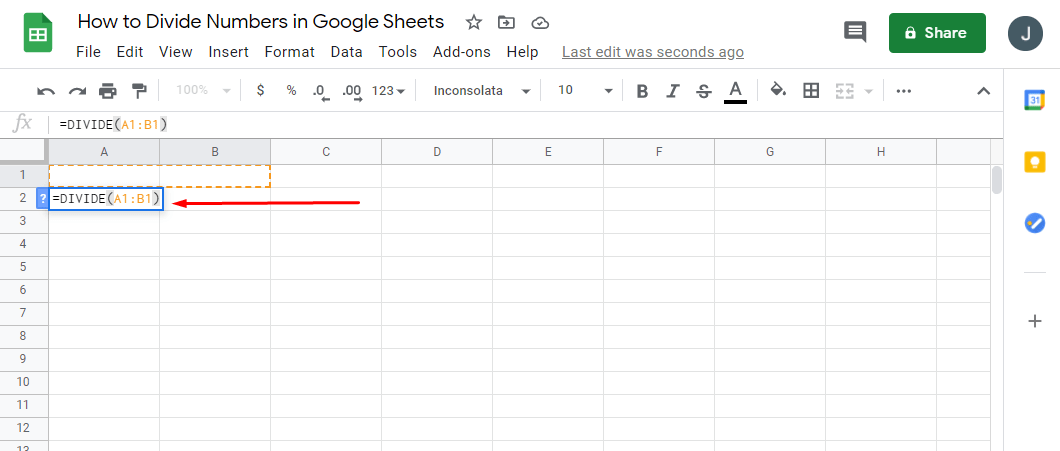• Using a multiply operator with numbers: “=2/3”• Using a multiply operation with cell names: “=B1/B2”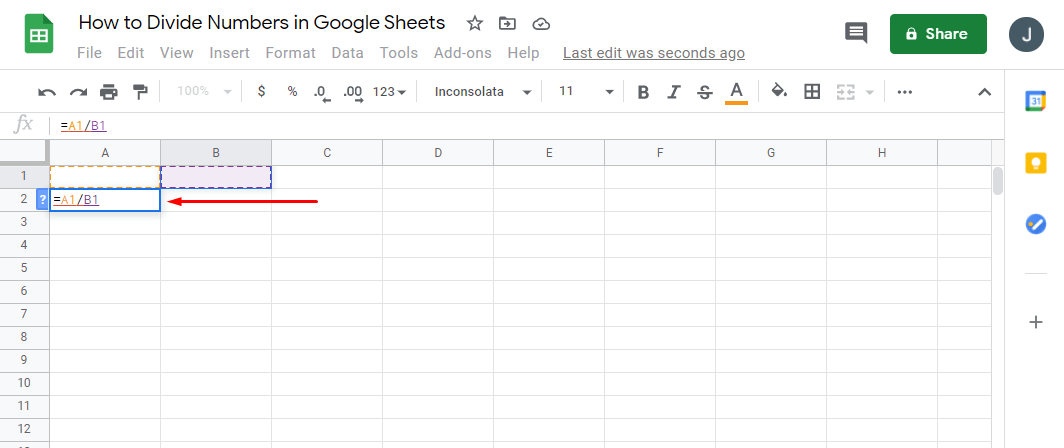## Sheets Are Everywhere

Once you figure out how a formula expands spreadsheet functionality, you’ll want to create as many of them as possible. When you’re aware of how useful functions really are, working in Google Sheets will become a lot easier.

Now that we’ve broken down some of the basic principles of multiplying in Google Sheets, you can use it to improve your spreadsheets and make them more professional. Plus, you now know more about multiplying, dividing, and using SUM and array formulas.

How often do you use Google Sheets? Are there any of these options you’ve never heard of before? Share your thoughts in the comments section below.

Disclaimer: Some pages on this site may include an affiliate link. This does not effect our editorial in any way.

Todays Highlights